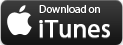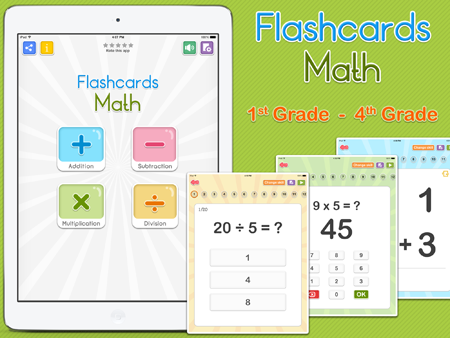The goals of Flashcards Math is practice basic addition, subtraction, multiplication and division. Each level is randomly generated. Features - Addition: 12 skills such as "Adding below six" - Subtraction: 14 skills such as "Subtraction below five" - Multiplication : 19 skills such as "Multiplication tables up to 12" - Division : 20 skills such as "Division facts for 1 to 5" - Various of flashcard style - Flashcard (Mark as "I know" and "I don't know" - Multiple Choice - Flll in the answer - Slideshow